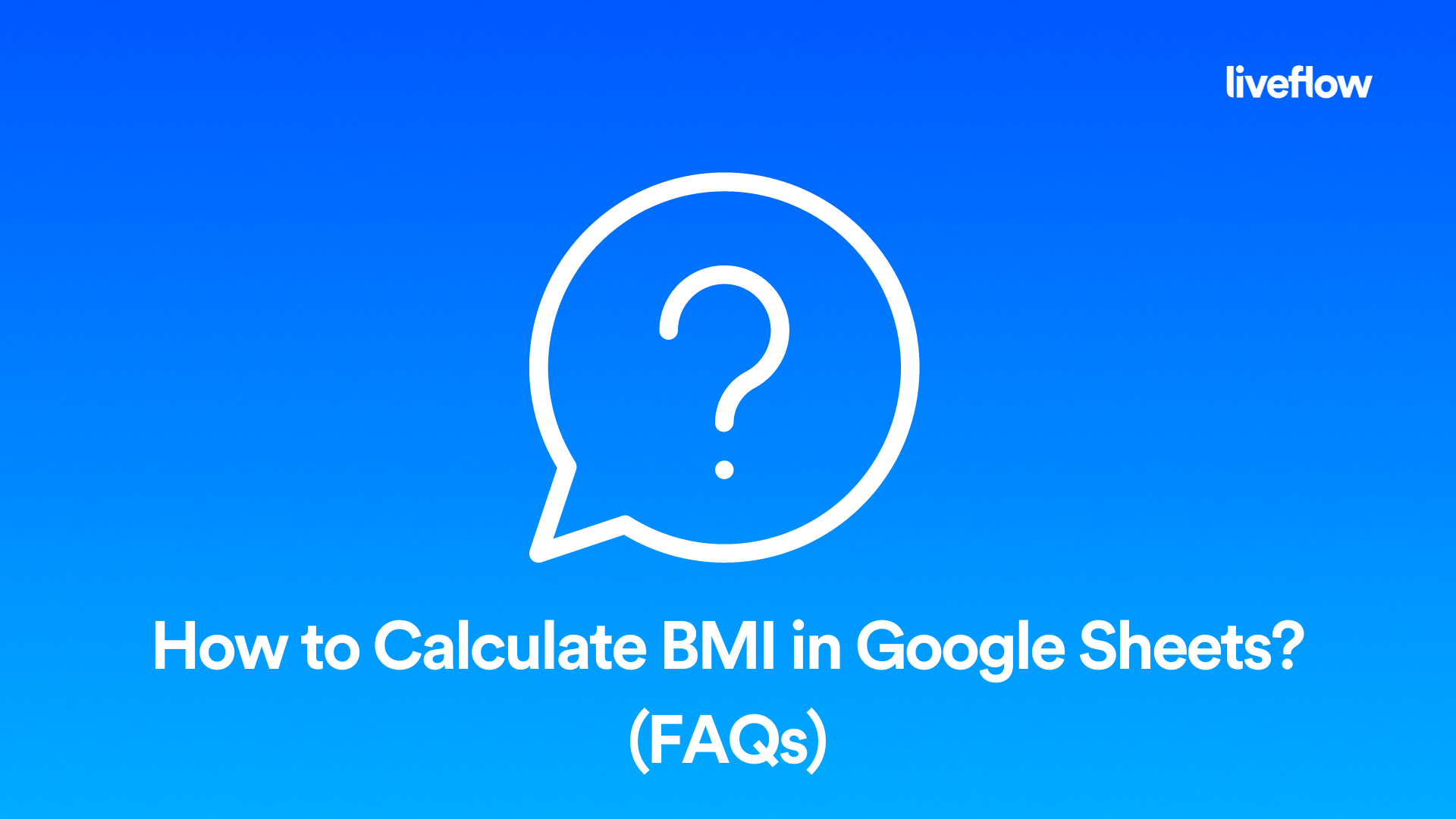# How to Calculate BMI in Google Sheets? (FAQs)

November 16, 2022You may have heard of body-mass index (BMI) and its usefulness as an indicator of whether you have a healthy weight. But perhaps you haven’t worked out your own personal BMI before and would like to do so using Google Sheets. Fortunately, it’s easy to calculate BMI in Google Sheets with only a few clicks.

In these FAQs we explain what BMI is and why we measure it. We also go over how to calculate BMI in Google Sheets.

## What is BMI?

BMI stands for ‘body-mass index’. It’s a simple way of working out whether you’re a healthy weight for your height. Your BMI is calculated by dividing your weight in kilograms by your height in metres squared. This is a very simple calculation that you can easily do with a spreadsheet such as Google Sheets.

There are some limitations to BMI as a measure of health. It is not accurate for people who are very muscular, or who are very thin. It also doesn’t take into account other factors that can affect your health, like how much exercise you do or what your diet is like. But it’s a simple and easy calculation to do, and it’s a helpful indicator of whether you’re a healthy weight.

## How to calculate BMI in google sheets

To calculate Body Mass Index (BMI) in Google Sheets, you can use the following formula:

BMI = weight/(height*height)

where weight is the weight in kilograms and height is the height in meters.

You can also use the following formula to calculate BMI in pounds and inches:

BMI = 703*weight/(height*height)

where weight is the weight in pounds and height is the height in inches.

To use the formula, simply enter it into a cell in your Google Sheets spreadsheet and replace weight and height with the appropriate cell references or values. For example, if you have the weight in kilograms in cell A1 and the height in meters in cell B1, you can use the following formula to calculate BMI:

BMI = A1/(B1*B1)

As you can see from the above, All you need to do is to type this into the Google Sheets spreadsheet and then fill in the ‘value’ with your own details. As mentioned above, if you want to calculate someone else’s BMI, for example a friend’s, you can do the same thing.

## How do you calculate BMI on a spreadsheet?

The simple formula for calculating BMI on a spreadsheet is: =weight / (height * height) Once again, all you need to do is to type this into the Google Sheets spreadsheet and then fill in the ‘value’ with your own details.

Check below for a visual guide:

## Does Google sheets have a BMI formula?

No, Google sheets does not have an in-built BMI formula. But the BMI formula can be typed manually into your spreadsheet and then filled in with your own details to work out your BMI.

## How do I insert a formula in Google Sheets?

1. Open the Google Sheets document where you want to insert the formula.
2. Click on the cell where you want to insert the formula. This will highlight the cell and make the formula bar visible at the top of the sheet.
3. Type the formula you want to use into the formula bar. For example, to insert a formula that adds the values in cells A1 and B1, you would type "=A1+B1" into the formula bar.
4. Press Enter or Return to complete the formula. The result of the formula will be displayed in the selected cell.

You can also insert a formula directly into a cell by clicking on the cell and typing the formula directly into the cell. Just make sure to start the formula with an equals sign (=).

If you need to reference a cell or range of cells in your formula, you can simply click on the cell or range of cells you want to reference. This will insert the cell reference into the formula for you.

For example, if you want to insert a formula that multiplies the value in cell A1 by the value in cell B1, you could click on cell A1, type an asterisk (), and then click on cell B1. This would insert the formula "=A1B1" into the formula bar, which would multiply the values in cells A1 and B1 when you press Enter or Return.

We hope these FAQs have helped you understand how to calculate BMI in Google Sheets.

## What is LiveFlow?

LiveFlow is a valuable platform built for QuickBooks, which is designed to make it easy to create an integrated, real time connection between your QuickBooks data and customized reports and dashboards in Google Sheets. This means that you don’t have to give anyone access to QuickBooks simply to review reports – you can use pre-built templates or bring live data to any customized report you already have.  This way, you only share what departments need to see. The live connection between QuickBooks and Google Sheets means that your reports will always be up to date – without any manual exports, data formatting or hands-on effort.

If you’d like to explore the potential of your financial data, we’d love to show you how. Contact the LiveFlow team to ask questions or  book a live demo.